## Introduction

R package corrplot provides a visual exploratory tool on correlation matrix that supports automatic variable reordering to help detect hidden patterns among variables.

corrplot is very easy to use and provides a rich array of plotting options in visualization method, graphic layout, color, legend, text labels, etc. It also provides p-values and confidence intervals to help users determine the statistical significance of the correlations.

corrplot() has about 50 parameters, however the mostly common ones are only a few. We can get a correlation matrix plot with only one line of code in most scenes.

The mostly using parameters include method, type, order, diag, and etc.

There are seven visualization methods (parameter method) in corrplot package, named 'circle', 'square', 'ellipse', 'number', 'shade', 'color', 'pie'. Color intensity of the glyph is proportional to the correlation coefficients by default color setting.

• 'circle' and 'square', the areas of circles or squares show the absolute value of corresponding correlation coefficients.

• 'ellipse', the ellipses have their eccentricity parametrically scaled to the correlation value. It comes from D.J. Murdoch and E.D. Chow’s job, see in section References.

• 'number', coefficients numbers with different color.

• 'color', square of equal size with different color.

• 'shade', similar to 'color', but the negative coefficients glyphs are shaded. Method 'pie' and 'shade' come from Michael Friendly’s job.

• 'pie', the circles are filled clockwise for positive values, anti-clockwise for negative values.

corrplot.mixed() is a wrapped function for mixed visualization style, which can set the visual methods of lower and upper triangular separately.

There are three layout types (parameter type): 'full', 'upper' and 'lower'.

The correlation matrix can be reordered according to the correlation matrix coefficients. This is important to identify the hidden structure and pattern in the matrix.

library(corrplot)
## corrplot 0.90 loaded
M = cor(mtcars)
corrplot(M, method = 'number') # colorful number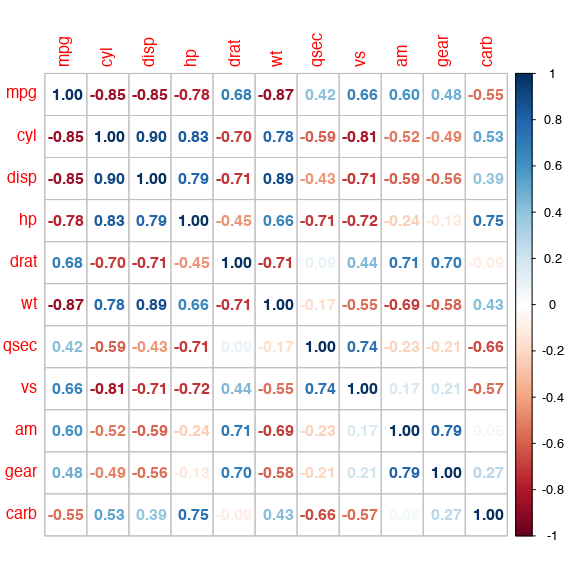corrplot(M, method = 'color', order = 'alphabet')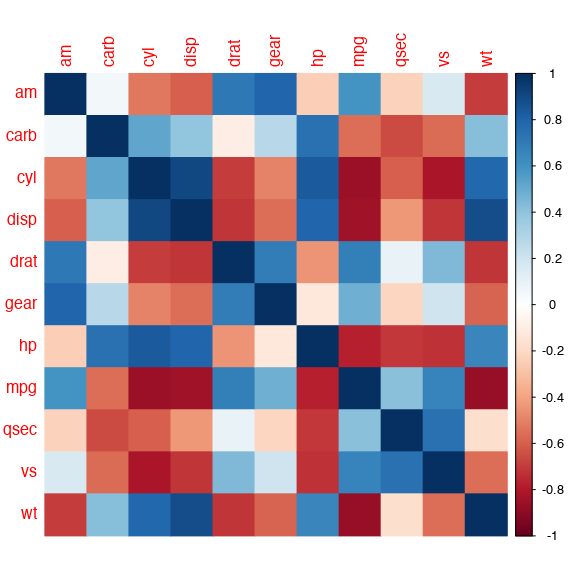corrplot(M) # by default, method = 'circle'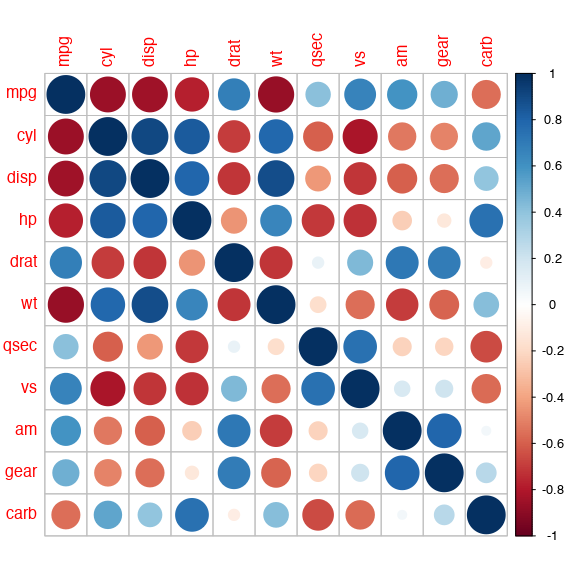corrplot(M, order = 'AOE') # after 'AOE' reorder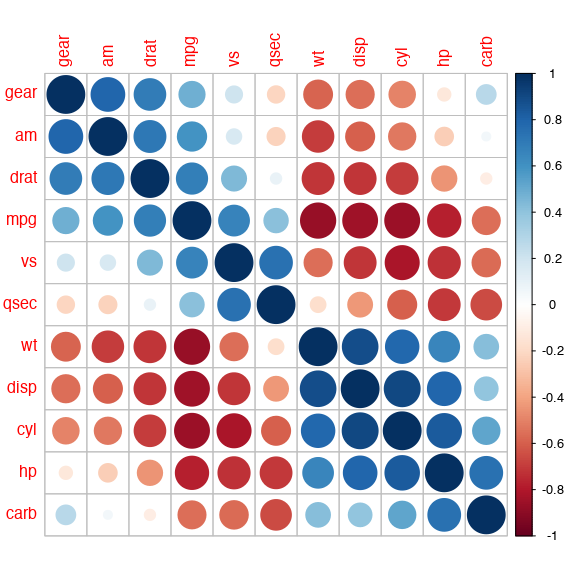corrplot(M, method = 'shade', order = 'AOE', diag = FALSE)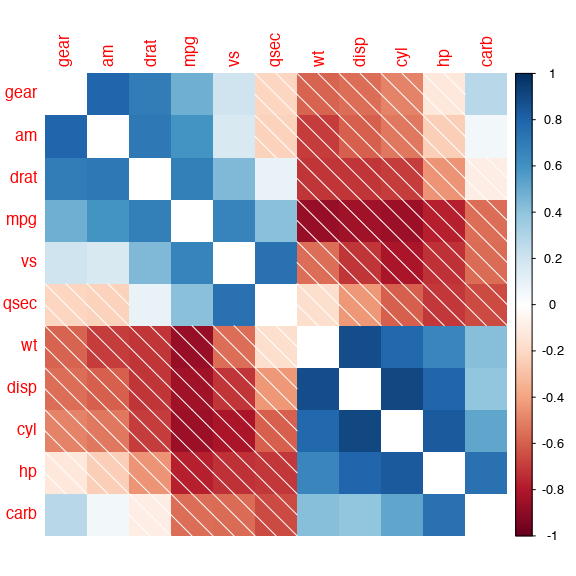corrplot(M, method = 'square', order = 'FPC', type = 'lower', diag = FALSE)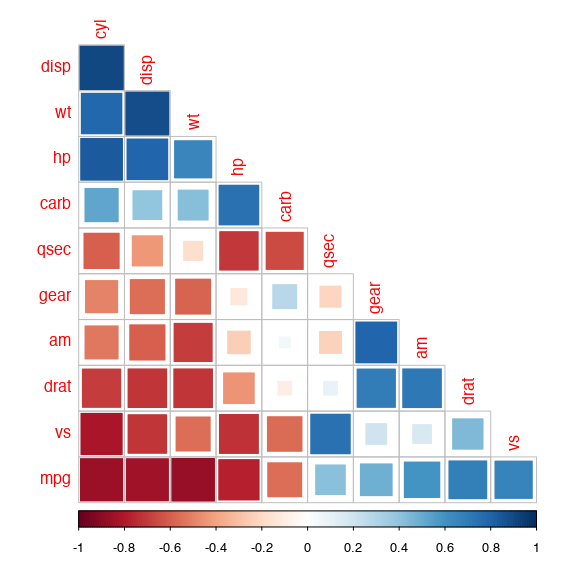corrplot(M, method = 'ellipse', order = 'AOE', type = 'upper')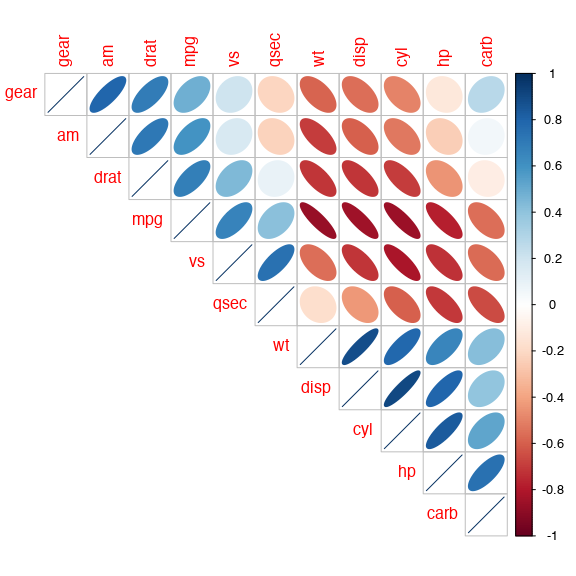corrplot.mixed(M, order = 'AOE')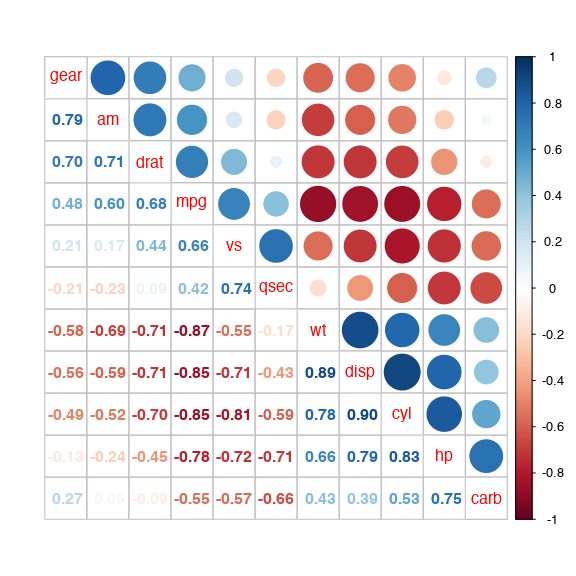corrplot.mixed(M, lower = 'shade', upper = 'pie', order = 'hclust')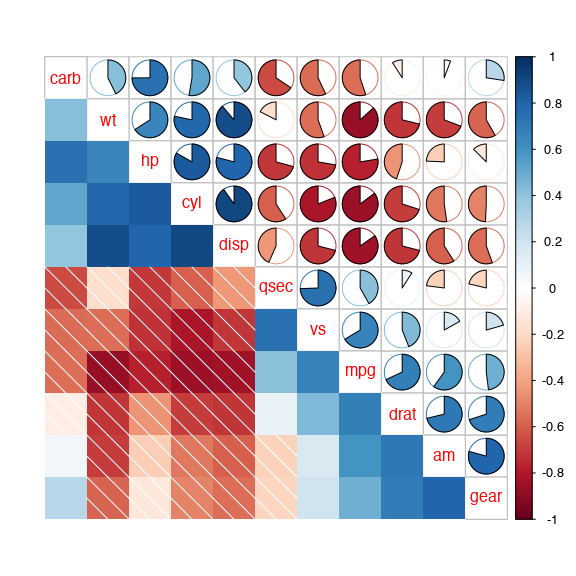## Reorder a correlation matrix

The details of four order algorithms, named 'AOE', 'FPC', 'hclust', 'alphabet' are as following.

• 'AOE' is for the angular order of the eigenvectors. It is calculated from the order of the angles $$a_i$$,

$a_i = \begin{cases} \arctan (e_{i2}/e_{i1}), & \text{if e_{i1}>0;} \newline \arctan (e_{i2}/e_{i1}) + \pi, & \text{otherwise.} \end{cases}$

where $$e_1$$ and $$e_2$$ are the largest two eigenvalues of the correlation matrix. See Michael Friendly (2002) for details.

• 'FPC' for the first principal component order.

• 'hclust' for hierarchical clustering order, and 'hclust.method' for the agglomeration method to be used. 'hclust.method' should be one of 'ward', 'single', 'complete', 'average', 'mcquitty', 'median' or 'centroid'.

• 'alphabet' for alphabetical order.

You can also reorder the matrix ‘manually’ via function corrMatOrder().

If using 'hclust', corrplot() can draw rectangles around the plot of correlation matrix based on the results of hierarchical clustering.

corrplot(M, order = 'hclust', addrect = 2)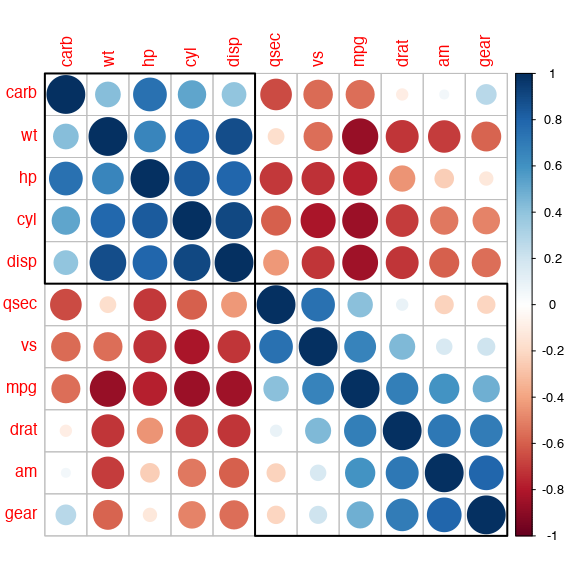corrplot(M, method = 'square', diag = FALSE, order = 'hclust',
addrect = 3, rect.col = 'blue', rect.lwd = 3, tl.pos = 'd')